Courses

# RD Sharma Solutions -Ex-6.1, Fractions, Class 6, Maths Class 6 Notes | EduRev

## RD Sharma Solutions for Class 6 Mathematics

Created by: Abhishek Kapoor

## Class 6 : RD Sharma Solutions -Ex-6.1, Fractions, Class 6, Maths Class 6 Notes | EduRev

The document RD Sharma Solutions -Ex-6.1, Fractions, Class 6, Maths Class 6 Notes | EduRev is a part of the Class 6 Course RD Sharma Solutions for Class 6 Mathematics.
All you need of Class 6 at this link: Class 6

Q1) Write the fraction representing the shaded portion :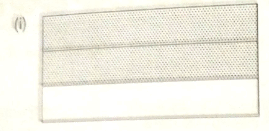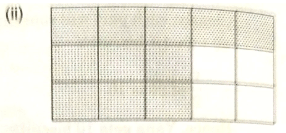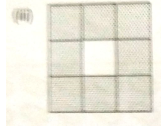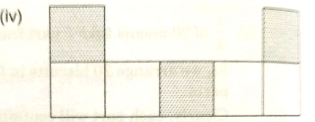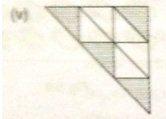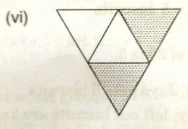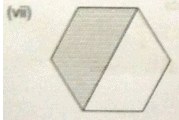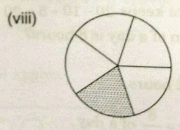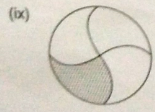Ans:

Fraction of the shaded portion = Number of shaded parts/Total number of parts

i) Total number of parts = 3

Number of shaded parts = 2

Fraction of the shaded portion = 2/3

(ii) Total number of parts = 15

Number of shaded parts = 11

Fraction of the shaded portion = 11/15

(iii) Total number of parts = 9

Number of shaded parts = 8

Fraction of the shaded portion = 8/9

(iv) Total number of parts = 7

Number of shaded parts = 3

Fraction of the shaded portion = 3/7

(v)Total number of parts = 9

Number of shaded parts = 4

Fraction of the shaded portion = 4/9

(vi)Total number of parts = 4

Number of shaded parts = 2

Fraction of the shaded portion = 2/4 = 1/2

(vii)Total number of parts = 2

Number of shaded parts = 1

Fraction of the shaded portion = 1/2

(viii)Total number of parts = 5

Number of shaded parts = 1

Fraction of the shaded = 1/5

(ix)Total number of parts = 4

Number of shaded parts = 1

Fraction of the shaded portion = 1/4

Q2) Write the fraction representing the shaded parts :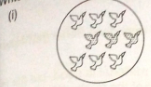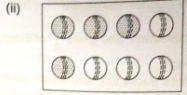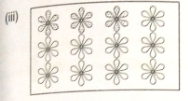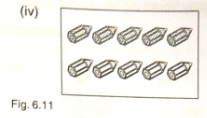Ans:

Fraction of the shaded portion = Number of shaded parts = Total number of parts

(i) Total number of parts = 9

Number of shaded parts = 3

Fraction of the shaded portion = 3/9 = 1/3

(ii) Total number of parts = 8

Number of shaded parts = 5

Fraction of the shaded portion = 4/8 = 1/2

(iii) Total number of parts = 12

Number of shaded parts = 3

Fraction of the shaded portion = 3/12 = 1/4

(iv) Total number of parts = 10

Number of shaded parts = 5

Fraction of the shaded portion = 5/10 = 1/2

Q3)Write the fraction representing the shaded portion :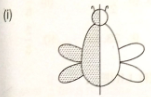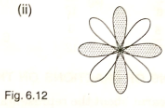Ans:

a) Total number of parts = 2

Number of shaded parts = 1

Total number of parts = 1/2

b) Total number of parts = 8

Number of shaded parts = 4

Total number of parts = 4/8

Q4)Colour the part according to the fraction given :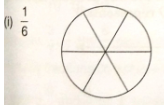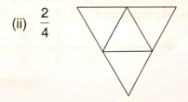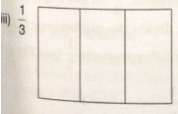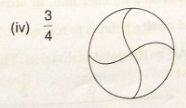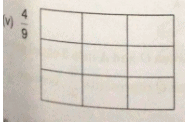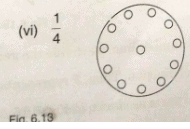Ans: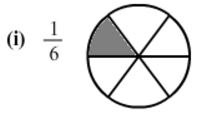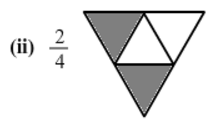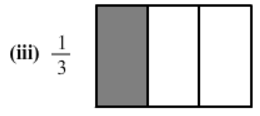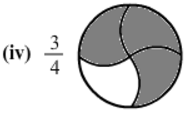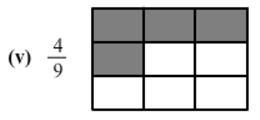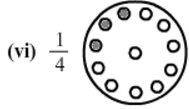Q5) What fraction of an hour is 20 minutes?

Ans. Minutes in an hour = 60

20 minutes of an hour = 2060 = 13

Q6) Write the natural numbers from 2 to 12. What fraction of them are prime numbers ?

Ans. Natural numbers from 2 to 12 are 2, 3 , 4 , 5 , 6 , 7 , 8 , 9 , 10 , 11 and 12.

Prime numbers from 2 to 12 are 2 , 3 , 5 , 7 and 11

Out of 11 numbers, 5 are prime.

Fraction of the prime numbers = 511

Q7) Write the natural numbers from 102 to 113. What fraction of them are prime numbers.

Sol :

Natural numbers from 102 to 113 are 102 , 103 , 104 , 105 , 106 , 107, 108 , 109 , 110 , 111 , 112 and 113.

Prime numbers from 102 to 113 are 103 , 107 , 109 and 113.

Out of 12 natural numbers , 4 are prime.

Fraction of the prime numbers = 412 = 13

Q8) Mukesh has a box of 24 pencils. He gives half of them to sunita. How many does sunita get ?

How many does mukesh still have?

Ans:

Given data: Mukesh has 24 pencils

Sunita gets half of mukesh’s pencils

Sunita gets 242 pencils, that is, 12 pencils.

Number of pencils mukesh still has = 24 – 12 = 12

Q9)Kavita has 44 cassettes. She gives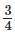of them to Sonia . How many does Sonia get? How many does kavita keep ?

Ans:

Kavita has 44 cassettes.

She gives 34 of the cassettes to Sonia.

For this, Kavita divides 44 cassettes in 4 equal parts and takes 3 parts.

Therefore,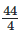= 11

It means that Kavita gives 33 cassettes to Sonia.

Number of cassettes Kavita has = 44 - 33 = 11

Q10) Shikas has three frocks that she wears when playing. The material is good, but the colours are faded. Her mother buys some blue dye and uses it on two of the frocks. What fraction of all of the shikas play frocks did her mother dye ?

Ans:

Total frocks shikha has = 3

Number of frocks dyed by shikha’s mother = 2

Fraction of the dyed frocks = 23

Therefore, shikha’s mother dyed 23 of shikha’s frocks.

103 docs

,

,

,

,

,

,

,

,

,

,

,

,

,

,

,

,

,

,

,

,

,

,

,

,

,

,

,

,

,

,

;